# Week 3: #BarBarPlots

Tutorial
Get with the Plot!
Data Visualization
R
Learn how (not) to visualise within-subjects data in ggplot2.
Author
Published

October 23, 2018

This is the third of a series of posts on how to use `ggplot2` to visualise data in R. This post introduces some new functions—use the `help()` if anything is unclear.

We begin by loading the `tidyverse` package which contains `ggplot2` alongside other useful packages. If you haven’t yet, you first need to install the `tidyverse` package by running `install.packages("tidyverse")`.

``library(tidyverse)``

This week’s dataset contains 160 observations of four variables. Each `person` has two observations of the `outcome` variable—one `before` and one `after` an unspecified event. This event could be, for example, an experimental manipulation or intervention.

Another variable divides people into two groups—on average, persons in one group score `low` on the outcome, while persons in the other tend to score `high` on the outcome. For now, we ignore the `group` variable.

``````dl <- read_rds("https://github.com/nilsreimer/data-visualisation-workshop/raw/master/materials/gwtp/dl_wk3.rds")
print(dl, n = 5)``````
``````# A tibble: 160 × 4
person group time   outcome
<int> <ord> <ord>    <int>
1      1 low   before      41
2      1 low   after       NA
3      2 low   before      30
4      2 low   after       20
5      3 high  before      55
# ℹ 155 more rows``````

This dataset represents within-subjects data in the so-called long format. That is, each row represents one of two observations for one of the 80 persons in this dataset. Note that some observations are missing (`NA`).

The long format is useful for visualising within-subjects data as `ggplot2` expects to find one row per data point. We set up our plot using the `ggplot()` function.

``````ggplot(dl, aes(x = time, y = outcome)) +
scale_y_continuous(limits = c(0, 100)) +
coord_fixed(1/25)``````This should all be familiar by now. Note that `ggplot()` recognises that `time` is an ordered categorical variable and represents it as such.

We use the `stat_summary()` function to create a bar plot.

``````ggplot(dl, aes(x = time, y = outcome)) +
stat_summary(geom = "bar", fun.y = "mean") +
scale_y_continuous(limits = c(0, 100)) +
coord_fixed(1/25)``````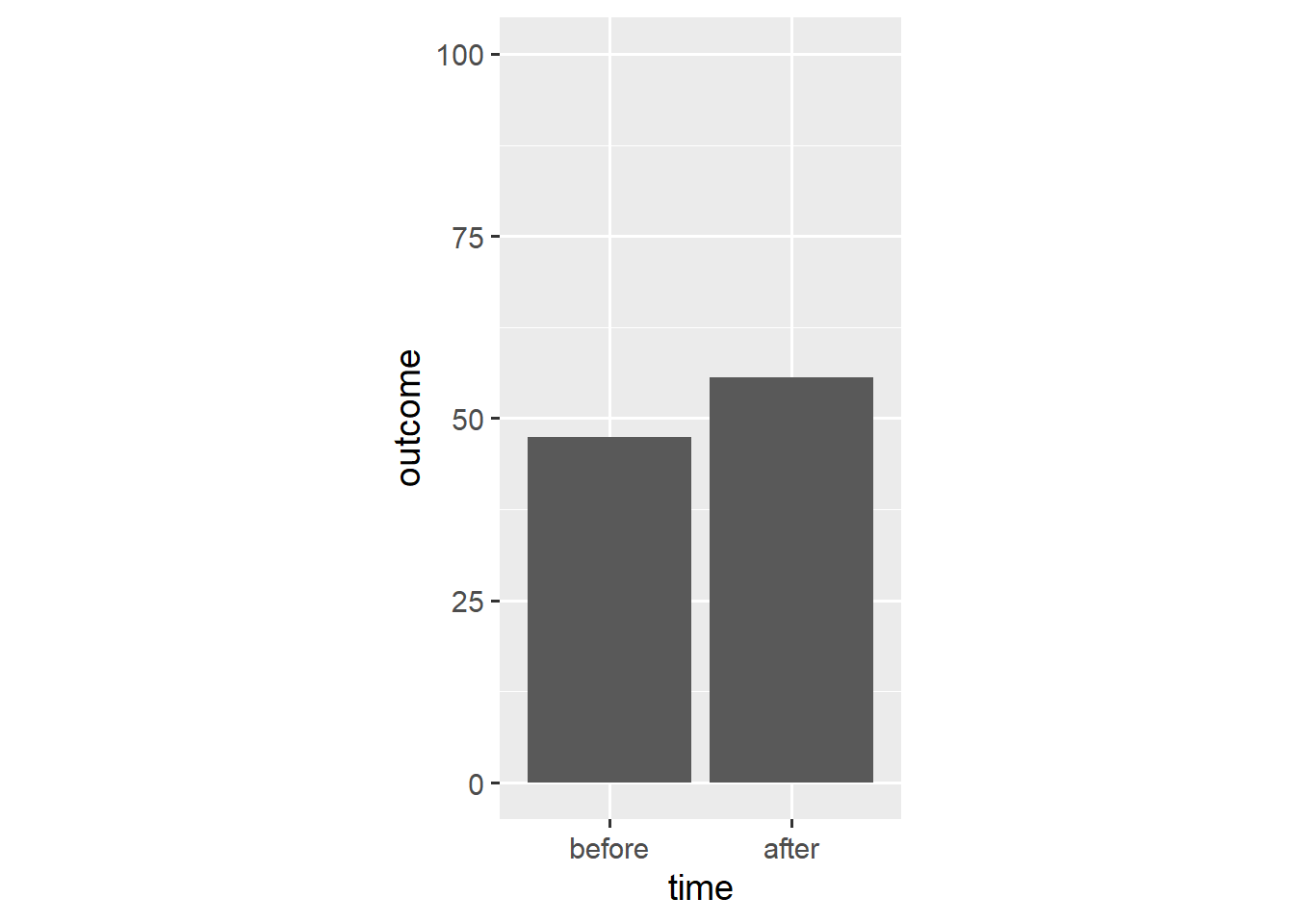The `stat_summary()` function aggregates data according to a specified function and represents the resulting aggregate with a specified geom. In this case, `stat_summary()` applies the `mean()` function to the `outcome` data and adds a `geom_bar()` layer that takes the resulting mean as its `y` aesthetic.

A bar plot is different from other visualisations we have used thus far. Rather than show each observation, a bar plot summarises all observations. As we will see, this often makes for a misleading visualisation.

For one, bar plots do not communicate the uncertainty around the underlying aggregate. We can remedy this shortcoming by adding error bars to our plot. We again use the `stat_summary()` function.

``````ggplot(dl, aes(x = time, y = outcome)) +
stat_summary(geom = "bar", fun.y = "mean") +
stat_summary(geom = "errorbar", fun.data = "mean_cl_boot",
width = 0.1) +
scale_y_continuous(limits = c(0, 100)) +
coord_fixed(1/25)``````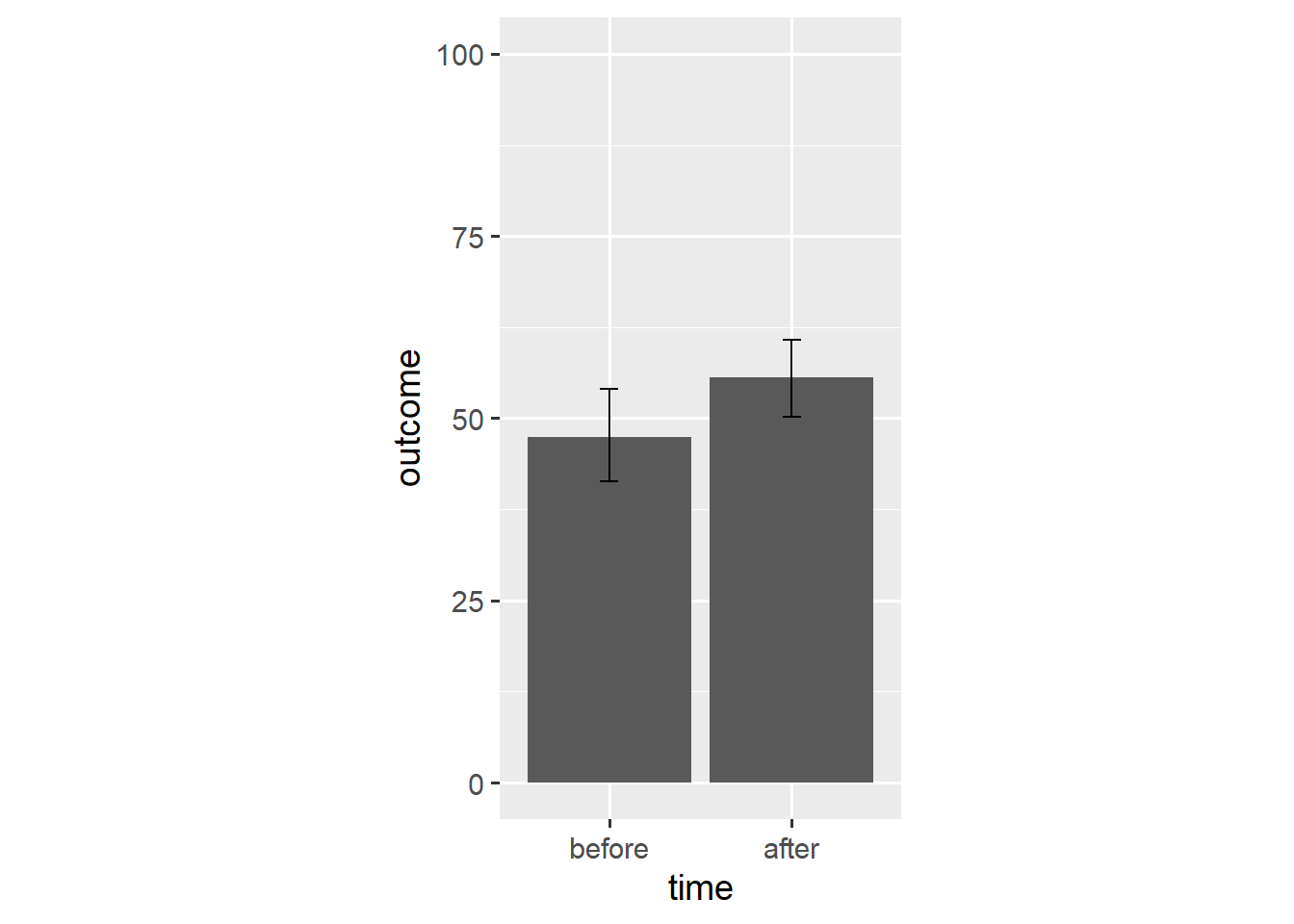In this case, the `mean_cl_boot()` function outputs a mean and its bootstrap confidence interval which provides the `ymin` and `ymax` aesthetics for a `geom_errorbar()` layer.

Instead, we can create a `geom_pointrange()` layer that shows both the point estimate and its confidence interval.

``````ggplot(dl, aes(x = time, y = outcome)) +
stat_summary(geom = "bar", fun.y = "mean") +
stat_summary(geom = "pointrange", fun.data = "mean_cl_boot",
shape = "diamond filled", fill = "white") +
scale_y_continuous(limits = c(0, 100)) +
coord_fixed(1/25)``````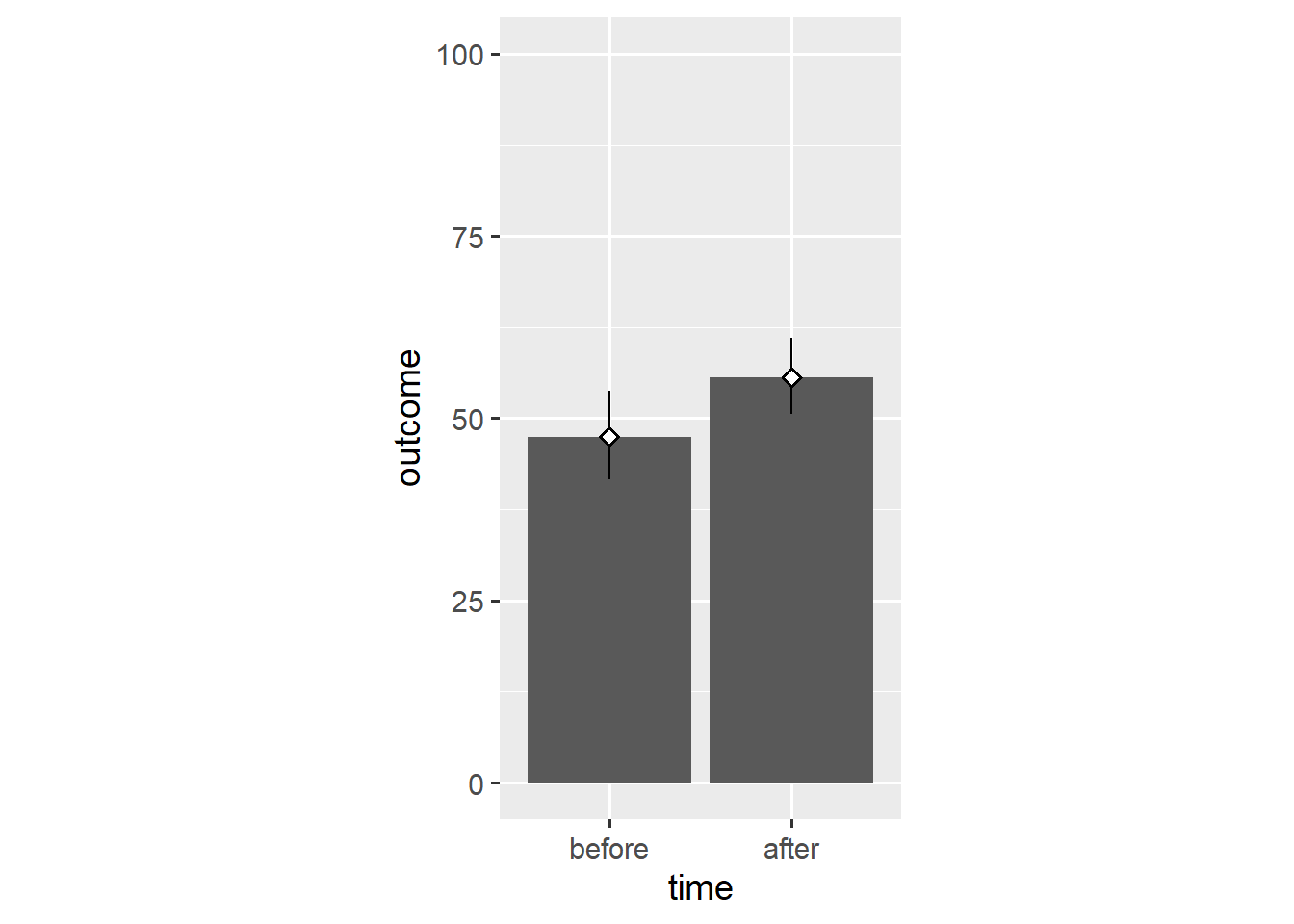Now the bars’ only unique function is to point towards `y == 0`. As such, we can replace them with a simple reference line.

``````ggplot(dl, aes(x = time, y = outcome)) +
geom_hline(yintercept = 0, colour = "white", size = 2) +
stat_summary(geom = "pointrange", fun.data = "mean_cl_boot",
shape = "diamond filled", fill = "white") +
scale_y_continuous(limits = c(0, 100)) +
coord_fixed(1/25)``````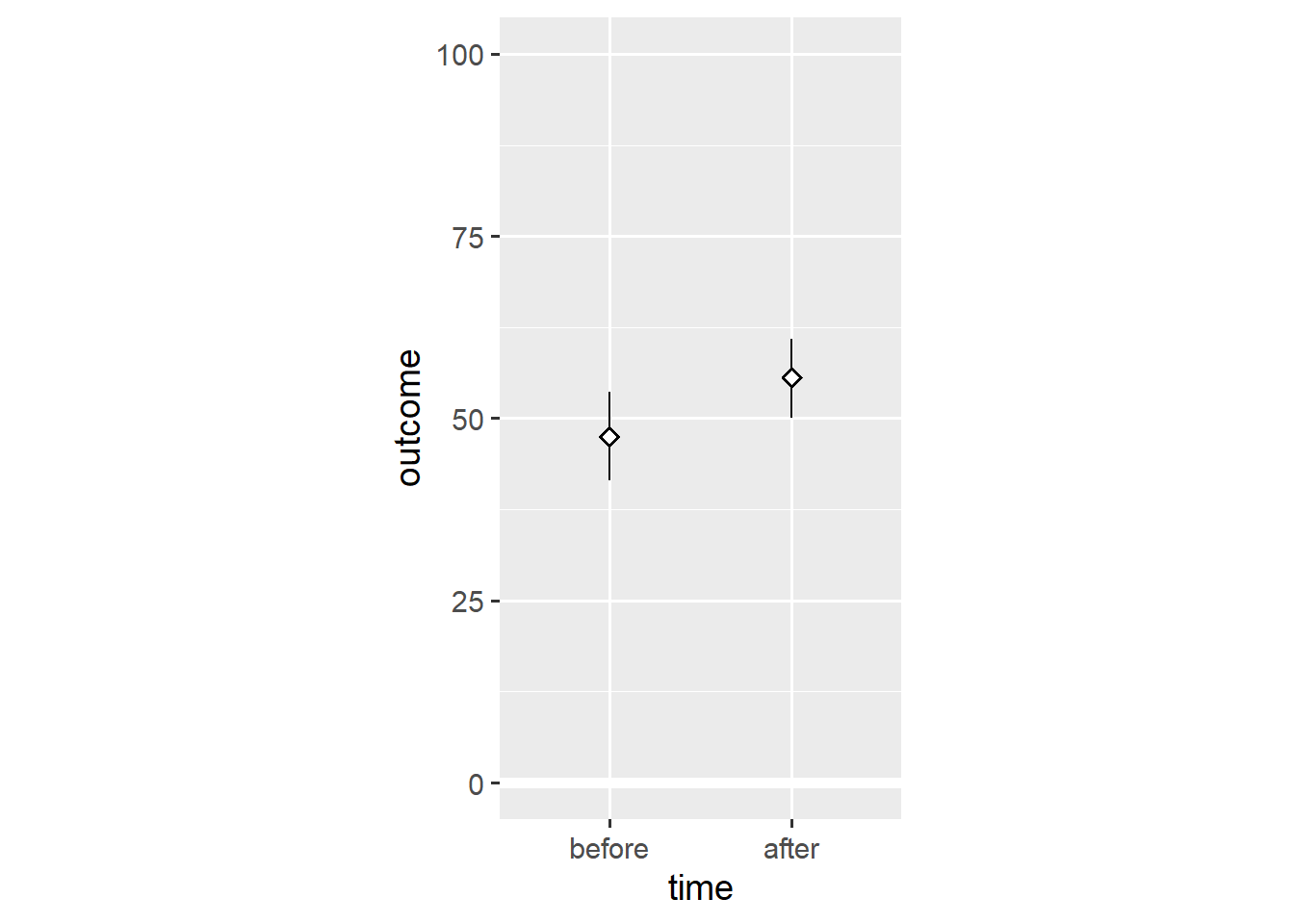Again, this shows that bar plots are summaries of the underlying data.1 Note that, based on the means, we would conclude that people tend to have higher `outcome` values `after` the relevant event.

In the first post, I made the point that `ggplot2` doesn’t constrain us to discrete types of plots, but provides a grammar for combining different elements of plots. As such, we can add individual data points to the plot.

``````ggplot(dl, aes(x = time, y = outcome)) +
geom_hline(yintercept = 0, colour = "white", size = 2) +
geom_point(colour = "grey70") +
stat_summary(geom = "pointrange", fun.data = "mean_cl_boot",
shape = "diamond filled", fill = "white") +
scale_y_continuous(limits = c(0, 100)) +
coord_fixed(1/25)``````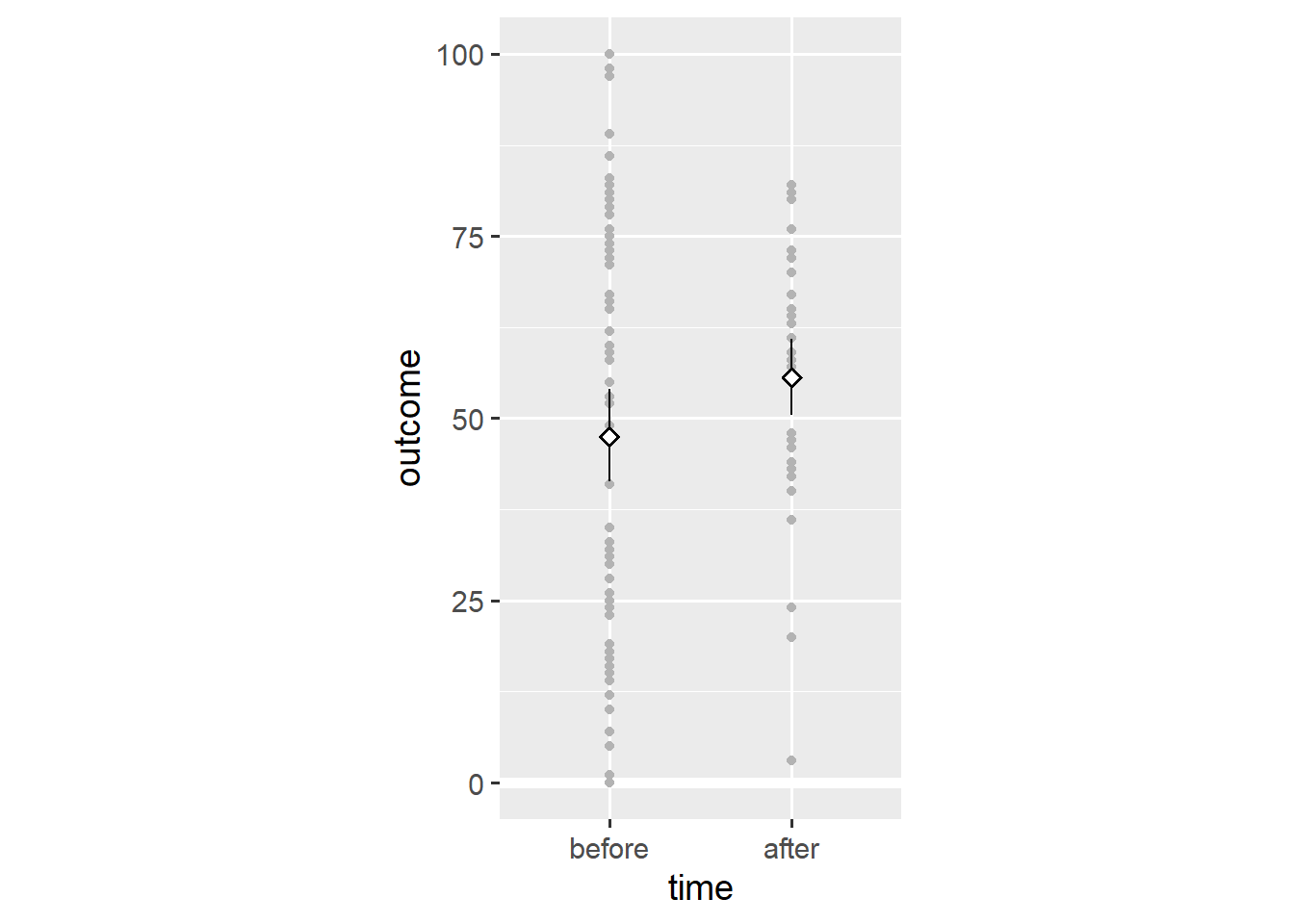Adding the `geom_point()` layer reveals the spread of the data. Still, this visualisation doesn’t consider that we’re dealing with within-subjects data—some observations come from the same person. We add lines that connect observations of the same `person` using the `group` aesthetic.

``````ggplot(dl, aes(x = time, y = outcome)) +
geom_hline(yintercept = 0, colour = "white", size = 2) +
geom_line(aes(group = person), colour = "grey70") +
geom_point(colour = "grey70") +
stat_summary(geom = "pointrange", fun.data = "mean_cl_boot",
shape = "diamond filled", fill = "white") +
scale_y_continuous(limits = c(0, 100)) +
coord_fixed(1/25)``````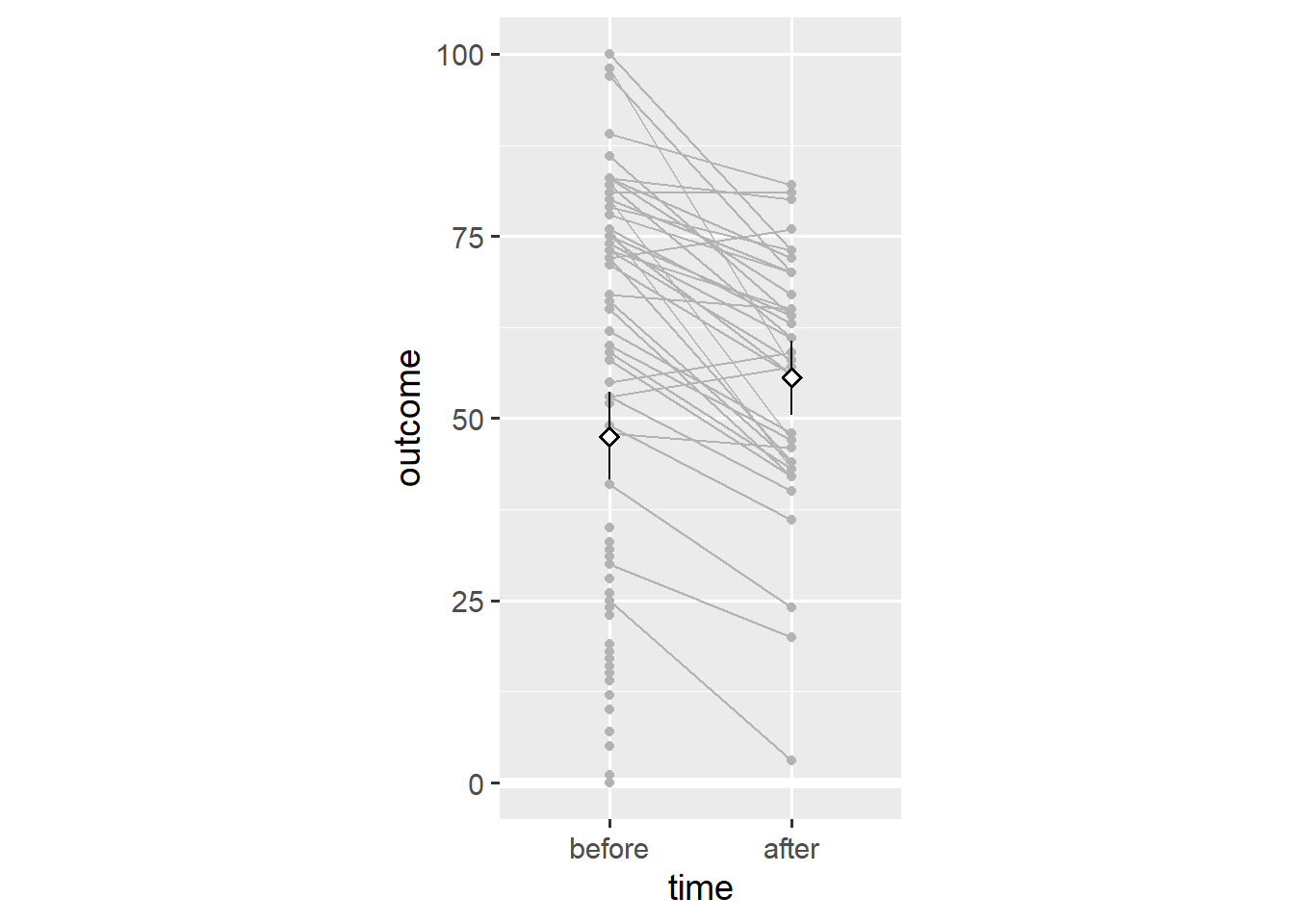Note that almost every person has a lower `outcome` value `after` the relevant event. This is in stark contrast to our earlier conclusion that people tend to have higher `outcome` values `after` the relevant event.

To understand what’s going on, we can use the `group` variable in the dataset (which distinguishes between people `low` and `high` on the `outcome` variable). We create distinct means for each `group` by using the `colour` aesthetic.

``````ggplot(dl, aes(x = time, y = outcome, colour = group)) +
geom_hline(yintercept = 0, colour = "white", size = 2) +
stat_summary(geom = "pointrange", fun.data = "mean_cl_boot",
shape = "diamond filled", fill = "white") +
scale_y_continuous(limits = c(0, 100)) +
coord_fixed(1/25)``````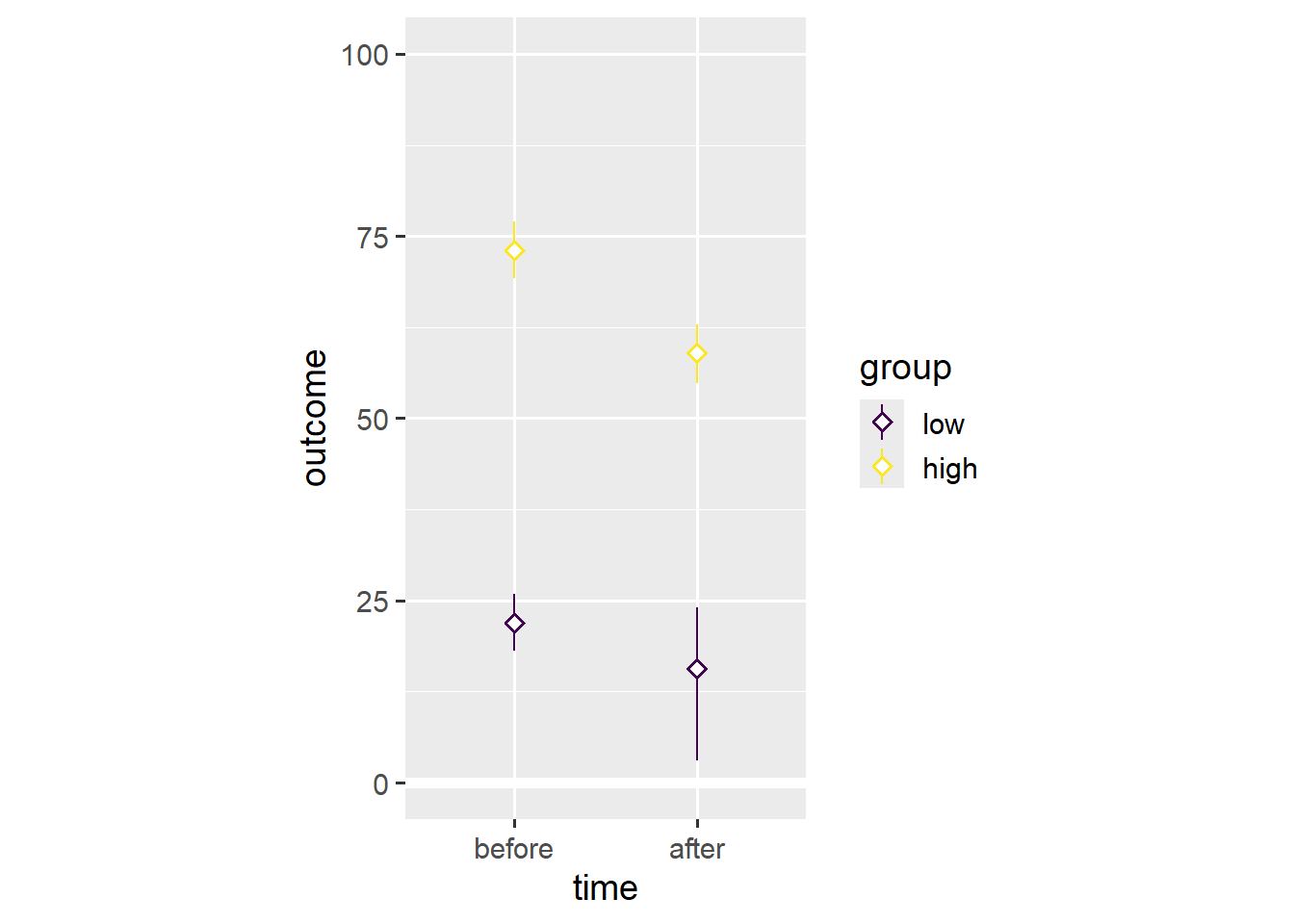Note that both groups have lower mean values after the event. This is an example of Simpson’s paradox in which a trend in different subgroups of the data disappears or reverses after combining the different subgroups.

We use the `colour` aesthetic to divide data points into the `low` and `high` subgroups.

``````ggplot(dl, aes(x = time, y = outcome, colour = group)) +
geom_hline(yintercept = 0, colour = "white", size = 2) +
geom_line(aes(group = person)) +
geom_point() +
scale_y_continuous(limits = c(0, 100)) +
coord_fixed(1/25)``````In this case, Simpson’s paradox resulted from differential dropout. Far more people with `low` initial values on the `outcome` variable seem to have dropped out before the second time point. As such, the average across the remaining people is higher `after` the relevant event, even though almost everyone’s `outcome` value has gone down.

Had we stuck with a bar plot, we wouldn’t have noticed. Presenting continuous data as a bar plot is misleading because it hides the distribution of the underlying data. Presenting within-subjects data as a bar plot is misleading because doing so hides individual trajectories in the underlying data.2

Above, we have already used one alternative to the bar plot. We introduce the within-subjects scatter plot as another alternative.3 First, we need to spread the dataset into the wide format wherein each row contains both `outcome` values for one `person`.

``````dw <- dl %>% spread(time, outcome)
print(dw, n = 5)``````
``````# A tibble: 80 × 4
person group before after
<int> <ord>  <int> <int>
1      1 low       41    NA
2      2 low       30    20
3      3 high      55    59
4      4 low       23    NA
5      5 high      53    40
# ℹ 75 more rows``````

Second, we create a scatter plot akin to the ones we’ve created in the first two posts.

``````ggplot(dw, aes(x = before, y = after)) +
geom_point() +
scale_x_continuous(limits = c(0, 100)) +
scale_y_continuous(limits = c(0, 100)) +
coord_fixed(1)``````Third, we add a reference line that marks all points for which `before == after`.

``````ggplot(dw, aes(x = before, y = after)) +
geom_abline(intercept = 0, slope = 1, linetype = "dashed") +
geom_point() +
scale_x_continuous(limits = c(0, 100)) +
scale_y_continuous(limits = c(0, 100)) +
coord_fixed(1)``````Note that we fixed the axis ratio to 1. This plot shows that three people have higher `outcome` values `after` the event, that one person has the same `outcome` value `after` the event, and that most people have lower `outcome` values `after` the event. This plot is useful because it shows both the overall trend and individual variation. Other than our earlier plot, this plot doesn’t show dropout.

And that’s it for this post. You now understand why you shouldn’t use bar plots to visualise within-subjects data—and what to use instead. If you have a question or found a mistake, please comment on Twitter or send me an email.

Next week, we’ll work with themes to prepare figures for publication.

## Footnotes

1. Count data are a special case where all we have is a summary. In this case, a bar plot might make sense.↩︎

2. Weissgerber et al. (2015) and Rousselet et al. (2017) present further arguments and examples, and highlight alternatives to the bar plot.↩︎

3. I thank Matti Vuorre for the inspiration.↩︎